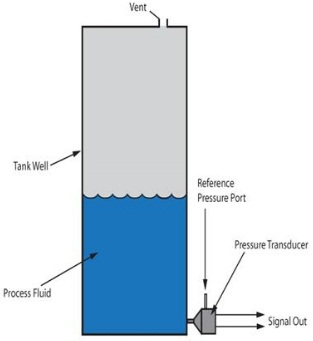# Basics of Hydrostatic level measurement## What is meant by hydrostatics?

Hydrostatic pressure sensors are used for the measurement of level or filling height of a liquid. Hydrostatic pressure measurement is suited for level measurement due to the hydrostatic effect of non flowing fluids. This physical principle describes the effect of the weight force of a stationary, meaning non-flowing, liquid on a measuring point. This weight force is usually described as “hydrostatic pressure”. The most important condition for hydrostatic level measurement is what is called the “hydrostatic paradox.” This means that, regardless of the shape and volume of a vessel, the hydrostatic pressure at the measuring point of a tank or vessel is proportional only to the filling height (Fig. ). Thus, despite the apparent contradiction of an over-proportional increase or decrease in the volume or weight of a liquid with change in height, the hydrostatic pressure at the measuring point is solely proportional to the absolute filling height, and not to the filling quantity.The hydrostatic pressure is not dependent upon the volume or the shape of a vessel

## Level measurement in open, vented vessels

In hydrostatic level measurement in open or vented basins or vessels, a continuous pressure compensation of the ambient air with the gas phase above the liquid takes place. Thus the ambient pressure that acts on the medium as an additional “force” always resembles the ambient pressure acting on the whole system, including the level sensor. If one therefore uses a pressure sensor with a relative pressure measuring cell, a pressure sensor that is compensated or vented (just like the tank) to the ambient pressure, it “automatically” compensates for the effect of this ambient pressure on the level measurement. This means that a relative pressure sensor in vented vessels and tanks completely “cancels out” the atmospheric pressure overlying on the liquid from the level measurement. Thus, the hydrostatic pressure corresponds only to the filling height of the liquid (Fig.). Therefore, the filling height of an open tank or vessel is calculated using the following equation:

h = p / (ρ * g)p = hydrostatic pressure [bar (relative)]

ρ = density of the liquid [kg/m³]

g = gravitational force or grav. acceleration [m/s²]

g = gravitational force or grav. acceleration [m/s²]

h = height of the liquid column [m]

## Level measurement in sealed, gas-tight vessels

The level measurement in sealed, gas-tight vessels, which is frequently found in the chemical industry, requires a compensation of the pressure of the enclosed gas phase above the liquid. The enclosed pressure of the gas phase acts as an additional force on the liquid and distorts any hydrostatic pressure measurement at the base of the vessel. Thus, this distorting influence must be compensated through an additional pressure measurement of the gas phase. Frequently, a second pressure sensor is used for the measurement of the gas pressure. This application effectively represents a differential pressure measurement, where the two separate pressure measurements are offset against each other (Fig. ). This offset calculation can be made either by two individual sensors or via an integrated differential pressure sensor. In this application, the sensors used can either be relative (sensor with ambient pressure compensation) or absolute pressure variants (sensor with sealed vacuum reference). Thus, the filling height of a sealed tank or vessel is calculated using the following equation:

h = (p2 – p1) / (ρ * g)p2 = hydrostatic pressure [bar]

p1 = pressure of the enclosed gas in the vessel [bar]

ρ = density of the fluid [kg/m³]

g = gravitational force or grav. acceleration [m/s²]

h = height of the liquid column [m]

## Advantages and limitations of hydrostatic level measurement

Hydrostatic pressure and level measurement enjoys a consistently high popularity due to it’s high robustness, high reliability and simple installation of this technology. The following characteristics constitute the greatest advantages and limitations over other measuring principles:

•  Proven and established measuring principle with high reliability, field-tested millions of times
• Robust measuring process, uninfluenced by disruptive factors such as dust, foam, vapour, buildup, contaminants, etc.
• Reliable measurement unaffected by many physical characteristics such as conductivity, dielectric coefficient or viscosity
• Level measurement unaffected by vessel geometry and existing installed equipment
• Simple installation and operation of submersible pressure transmitters and conventional pressure sensors without the need for calibration or adjustment
• Direct contact with the medium
• Numerous alternative design variations and sensor technologies for almost every application

### Limitations:

•  Unsuitable for bulk material
• Accurate measurement requires either media with constant density or continuous density measurement of the medium